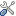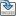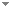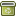# Varieties of Commutative Integral Bounded Residuated Lattices Admitting a Boolean Retraction Term

Studia Logica 100 (6):1107-1136 (2012)

 Abstract Let ${\mathbb{BRL}}$ denote the variety of commutative integral bounded residuated lattices (bounded residuated lattices for short). A Boolean retraction term for a subvariety ${\mathbb{V}}$ of ${\mathbb{BRL}}$ is a unary term t in the language of bounded residuated lattices such that for every ${{\bf A} \in \mathbb{V}, t^{A}}$ , the interpretation of the term on A, defines a retraction from A onto its Boolean skeleton B(A). It is shown that Boolean retraction terms are equationally definable, in the sense that there is a variety ${\mathbb{V}_{t} \subsetneq \mathbb{BRL}}$ such that a variety ${\mathbb{V} \subsetneq \mathbb{BRL}}$ admits the unary term t as a Boolean retraction term if and only if ${\mathbb{V} \subseteq \mathbb{V}_{t}}$ . Moreover, the equation s(x) = t(x) holds in ${\mathbb{V}_{s} \cap \mathbb{V}_{t}}$ . The radical of ${{\bf A} \in \mathbb{BRL}}$ , with the structure of an unbounded residuated lattice with the operations inherited from A expanded with a unary operation corresponding to double negation and a a binary operation defined in terms of the monoid product and the negation, is called the radical algebra of A. To each involutive variety ${\mathbb{V} \subseteq \mathbb{V}_{t}}$ is associated a variety ${\mathbb{V}^{r}}$ formed by the isomorphic copies of the radical algebras of the directly indecomposable algebras in ${\mathbb{V}}$ . Each free algebra in such ${\mathbb{V}}$ is representable as a weak Boolean product of directly indecomposable algebras over the Stone space of the free Boolean algebra with the same number of free generators, and the radical algebra of each directly indecomposable factor is a free algebra in the associated variety ${\mathbb{V}^{r}}$ , also with the same number of free generators.A hierarchy of subvarieties of ${\mathbb{BRL}}$ admitting Boolean retraction terms is exhibited Keywords Residuated lattices  Boolean products  Boolean retraction terms  Free algebras Categories Logic and Philosophy of Logic (categorize this paper) ISBN(s) DOI 10.1007/s11225-012-9453-4 OptionsEdit this recordMark as duplicateExport citationFind it on ScholarRequest removal from indexRevision history

PhilArchive copy

Upload a copy of this paper     Check publisher's policy     Papers currently archived: 71,259

Setup an account with your affiliations in order to access resources via your University's proxy server
Configure custom proxy (use this if your affiliation does not provide a proxy)